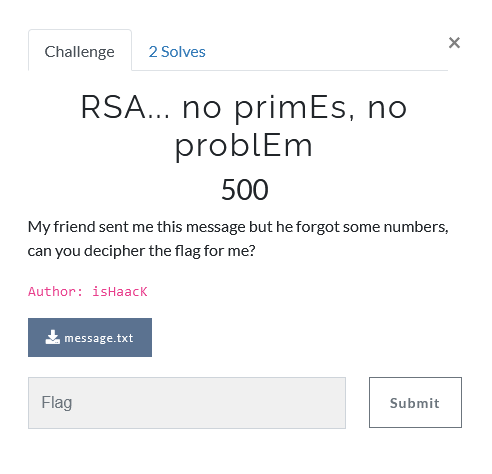# NetOn CTF 2021 - RSA... no primEs, no problEm

Cryptography – 500 pts (2 solves) – Chall author: isHaacK

Given both the public key and the secret value phi(n) we can derive the private component. There is one catch though, we do not know the public exponent… but we can just try until we find the right value.

## ChallengeAnother RSA challenge! But this time, the numbers are substantially bigger :c The challenge provided us with an encrypted flag, public component ‘n’ and phi(n).

ciphertext = 4803486620985796010075216068877609455871383897640323954843756796800173149437251687733347664192572117005126499615124844328373675595265268457208063073063362666296732488986704487923437514493478565848183238995971938189096941670496102571815095353480403637698691345512049769785098395967252710672386044217321956851169565136849198146328368935680111095304534054866044961612091553409953330964655033765906224029250112390318273915843693588625005937293557999267431911756800430138791635421069977

n = 14243345730631857177062718957286458295344875661901393577745661535671731182469817848719170841079560124200634261984946297612967896241817034756539493737449488275719622652895734552547316101216424483474862911698249829751472886166538635034783417821365701447757997511751589750140515711946334390780520161779493842518544535120769290658501358009251342540552113786916173497963072314840842823842101868020523794699586378835932131301349396908327821519900075538365718554522120543219846421981750367

phi(n) = 14243345730631857177062718957286458295344875661901393577745661535671731182469817848719170841079560124200634261984946297612967896241817034756539493737449488275719622652895734552547316101216424483474862911698249829751472886166538635034783417813763312676513662599184910888148679300475389478079315732593163367616609472566305826936916314217502538829723285645468007039496239828709581450294608647853663479553932636891934128772821535614594114832137463330741228691150099997156720502848674840


## Solution

Note that this time, ‘n’ is too large for us to factorise it. Even factordb cannot help us here. However, we are given a phi(n)… which we can use to derive the private component ‘d’ using d = ~e mod phi(n). There is one catch though, we do not know ‘e’… Luckily, for computational reasons, ‘e’ is usually not too big and we know it must be a coprime of phi(n), so we can use our previously used Euler inverse function. Now we just brute-force our way by incrementing a test value for ‘e’ and checking whether or not the output makes sense :). To do this, I used some Python again (I left out the flag, n, phi_n and eulinv() definitions)

itere = 3 # trial 'e'
while itere < int(1e6):
# Euler inverse (see previous RSA challenge)
d = eulinv(itere,phi_n)
# If d returned an actual value (i.e. e is a coprime)
if not d is None:
# Set RSA components
e = itere
d = eulinv(e, phi_n)
# Get message
mint = pow(flag,d,n)
mbit = bin(mint)[2:];
while len(mbit)%8 != 0:
mbit = '0' + mbit
m = [int(mbit[i:i+8],2) for i in range(0,len(mbit),8)]
# Check whether all message characters are within common ASCII range
if (min(m) >= 33) & (max(m) <= 125):
flag = ''.join([chr(i) for i in m])
print(e, flag)
break
# Increment 'e'
itere += 1


After a few seconds of thinking, it finds our flag at an ‘e’ value of 15049!

NETON{RSA_1s_r34lly_fun!}